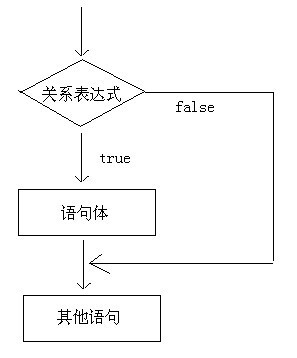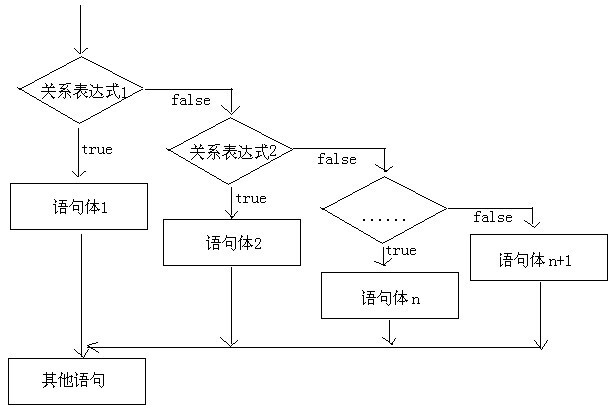Java 流程控制语句 - 分支结构 (选择结构)，java 技术专家面试题

• 2021 年 11 月 10 日
• 本文字数：1170 字

阅读完需：约 4 分钟

if 分支结构

第一种格式

if(条件表达式){

}

执行流程举例

public class IfDemo {

public static void main(String[] args) {

System.out.println("开始");

// 定义两个变量

int a = 10;

int b = 20;

if (a == b) {

System.out.println("a 等于 b");

}

int c = 10;

if (a == c) {

System.out.println("a 等于 c");

}

System.out.println("结束");

}

}

第二种格式

if(条件表达式){

}else{

}

执行流程

![if 语句格式 2](https://imgconvert.csdn

《Android学习笔记总结+最新移动架构视频+大厂安卓面试真题+项目实战源码讲义》

img.cn/aHR0cHM6Ly9yYXcuZ2l0aHVidXNlcmNvbnRlbnQuY29tL0pvdXJXb24vaW1hZ2UvbWFzdGVyL0phdmElRTUlOUYlQkElRTclQTElODAlRTglQUYlQUQlRTYlQjMlOTUvaWYlRTglQUYlQUQlRTUlOEYlQTUlRTYlQTAlQkMlRTUlQkMlOEYyLmpwZw?x-oss-process=image/format,png)

举例

public class IfDemo2 {

public static void main(String[] args) {

System.out.println("开始");

// 判断给定的数据是奇数还是偶数

// 定义变量

int a = 100;

// 给 a 重新赋值

a = 99;

if (a % 2 == 0) {

System.out.println("a 是偶数");

} else {

System.out.println("a 是奇数");

}

System.out.println("结束");

}

}

第三种格式

格式

if(条件表达式){

}else if(条件表达式){

}…(此处省略 N 个 else if)

else{

}

执行流程举例

public class IfDemo3 {

public static void main(String[] args) {

// x 和 y 的关系满足如下：

// x>=3 y = 2x + 1;

// -1<=x<3 y = 2x;

// x<=-1 y = 2x – 1;

// 根据给定的 x 的值，计算出 y 的值并输出。

// 定义变量

int x = 5;

/*

int y;

if (x >= 3) {

y = 2 * x + 1;

} else if (x >= -1 && x < 3) {

y = 2 * x;

} else if (x <= -1) {

y = 2 * x - 1;

}else {

y = 0;

}

*/

int y = 0;

if (x >= 3) {

y = 2 * x + 1;

} else if (x >= -1 && x < 3) {

y = 2 * x;

} else if (x <= -1) {

y = 2 * x - 1;

}

System.out.println("y 的值是："+y);

}

}

评论